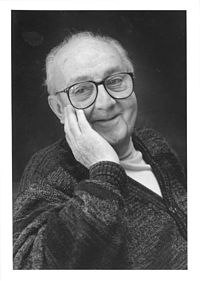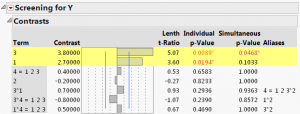Celebrating statisticians: George E.P. Box

In this International Year of Statistics, we at JMP are celebrating famous statisticians on a monthly basis. This month is my turn, and early this year I chose Professor George E.P. Box as the subject of my celebration. I was looking forward to writing this piece because I knew George personally and have been an admirer of his since the beginning of my career.Sadly, George passed away in late March, and I wrote a remembrance of him for the JMP Blog at that time. That blog post expresses what I would have written in a post celebrating him. So, instead of speaking in general about his life and accomplishments, in this post I will focus on one of his many great papers. My plan is to write several such blog posts this month, each emphasizing a different one of his wonderful publications. One of the benefits for me is that I get to reread these papers.

In this post, I want to focus on the first of his two-part paper with J. Stuart Hunter on the family of regular two-level fractional factorial designs that was published in Technometrics in 1961.

This seminal paper is 40 pages long, and one thing I found notable about it was that the mathematical content did not go past arithmetic and a little algebra! Despite this, there are many fundamental results in this paper, but all are stated in natural language without formal proofs. That was refreshing.

How does the paper begin?

The paper starts with a brief exposition of two-level full factorial design in k factors. It shows how these designs can estimate interactions of all orders up to the k-factor interaction. This provides the motivation and background for introducing a half fraction of the full factorial design. They illustrate the construction method using the 2(4-1) design showing how one starts with the full factorial design in three factors and then adds a fourth factor by computing the elementwise product of the first three factors.

Can you show this in JMP?

To reconstruct their example the way they did it by using JMP, we start by using the Full Factorial designer. We call our factors 1, 2 and 3 and our response Y. We compute the 4th column using the formula editor. The Y column in our Table 1 below has the same values as the ones they use in their Table 3.

Of course, we could also just use the Screening Designer in JMP to enter 4 factors. The design we want is the first in the list. :-)

What happens next?

They now have a design with 8 runs that is just half as many runs as are in the full factorial design with four factors. With the full factorial design, you can estimate 16 effects – the overall average, 4 main effects, 6 two-factor interactions, 4 three-factor interactions and 1 four-factor interaction (16 = 1 + 4 + 6 + 4 + 1). Now with 8 runs, you can only estimate 8 effects. It turns out that the construction the authors use confounds the 16 effects of the full factorial into 8 pairs of effects. The average is confounded with the four-factor interaction. The 4 main effects are each confounded with one of the 4 three-factor interactions. Finally, the 6 two-factor interactions are confounded in 3 pairs (8 = 1 + 4 + 3).

Figure 1 below shows the analysis from the JMP Screening platform. The values JMP reports are half of the quantities Box and Hunter report, because they define their effects as being the difference in the response when changing from one level of the factor to the other. JMP defines an effect as the change in the response due to a one-unit change in the factor. Since one level of the factor is coded -1 and the other is coded +1, each factor changes by two units going from its low to its high level. Thus, the effect of a one-unit change is half the effect of going from low to high.How does the rest of the paper go?

Of course, the paper is much too long for me to cover everything Box and Hunter introduce – especially not in this level of detail. Here are some of the big concepts:

• Generalizing their 4-factor example, they show that the best way to create a half fraction of a k factor full factorial design is to start with a full factorial design in k – 1 factors and then calculate the last column by computing the elementwise product of the original k – 1 columns. They also show that one can reconstitute the full factorial by combining a half fraction with another half fraction that where every value in the second fraction is obtained by multiplying the corresponding value in the first fraction by –1. This leads to the concept of a foldover design – a term they also introduce here.
• They introduce the idea of design resolution and define resolution III, IV and V designs. Introducing the idea of a saturated design, they describe resolution III designs of 7 factors in 8 runs, 15 factors in 16 runs and 31 factors in 32 runs. They also throw a bone to Plackett and Burman (1946) mentioning their constructions of 11 factors in 12 runs, 19 factors in 20 runs, 23 factors in 24 runs, etc.
• They introduce the idea of design generators and use this idea to show how to block the fractional factorial designs in groups of runs that each have 2, 4, 8 or some other power of 2 runs per block.
• They show how to obtain designs of resolution IV by folding over a design of resolution III and introduce the idea of design projectivity. For example, they state that every resolution IV design projects to a full factorial (or replicated full factorial) in any three of the factors. The benefit of this is that if only three factors turn out to be important, it is possible to estimate all the interaction effects of those three factors. And, it does not matter which three are important.
• Where has design for screening gone in the 50+ years since then?

It is a tribute to the combined power and simplicity of this approach that the regular two-level fractional factorial designs are still in frequent use today. The construction and analysis of these designs does not require a computer, which made them popular when computers were rare. Of course, the calculations can be a bit tedious, so having a computer do them for you makes for fewer errors and more free time.

In the same year as the publication of this paper, Hall published 5 different orthogonal arrays for 15 factors in 16 runs. The saturated design in the Box and Hunter’s paper was one of the 5. This paper was also fundamental as it turns out that all the orthogonal arrays 16 runs for fewer factors are projections of the Hall arrays.

Forty years later, Sun, et al. (2002) catalogued all the orthogonal 16 run designs for 5 to 14 factors. For 9 to 14 factors, the 16 run designs of Box and Hunter are all of resolution III, which means that main effects are confounded with two-factor interactions. Sun, et al. found designs in these cases where none of the two-factor interactions confounds a main effect. Instead, some two-factor interactions may be correlated either plus or minus one-half with a main effect. The benefit of these designs is that main effects can be identified without the built-in ambiguity that resolution III designs entail.

References

Box, G. E. P. and Hunter, J. S. (1961) "The 2k-p Fractional Factorial Designs Part I" Technometrics Vol 3, No. 3 311-351.

Hall, M. Jr. (1961). Hadamard matrix of order 16. Jet Propulsion Laboratory Research Summary, 1, 21–26.

Sun, D. X., Li, W., and Ye, K. Q. (2002), “An Algorithm for Sequentially Constructing Non-Isomorphic Orthogonal Designs and Its Applications,” Technical Report SUNYSB-AMS-02-13, State University of New York at Stony Brook, Dept. of Applied Mathematics and Statistics.

1 Comment
Community Member

Celebrating statisticians II: George Box and the Box-Behnken Designs - JMP Blog wrote:

[...] month. Professor George E.P. Box is the honoree for May. Last week, I wrote about on the first of his two-part paper with J. Stuart Hunter on the family of regular two-level fractional factorial designs that was [...]